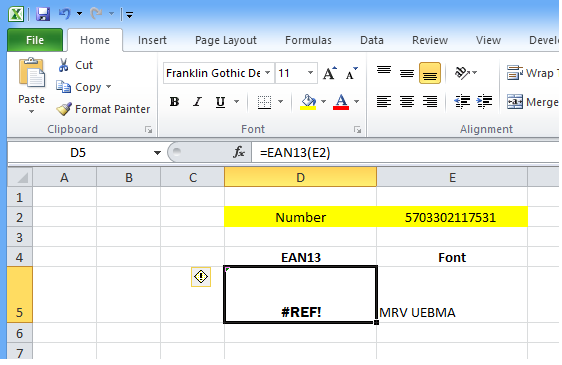Search For Category All ArticlesBarcode Fonts    General    Crystal Reports    PCL Fonts    Reporting Service    ProgrammingBarcode ComponentsLabeling SoftwareBarcode Knowledge    Symbology SpecificationsMorovia Web Site

## SYMPTOMS

You imported Font Tools VBA module following the instructions at Tutorial: Using Barcode Fonts in Excel Spreadsheets. After you put formula `=EAN13(E2)` (here `E2` is the reference to data cell), Excel gives #REF! error.## CAUSE

Excel 2010 increases the number of columns that it can address to 3-letter column names. As a result, names like `EAN13` are treated as a cell reference: column `EAN` and row `13`. The VBA function is never invoked.

## RESOLUTION

There are two solutions to this name confliction issue.

• Method I: Save the file in legacy Excel format (.xls), which is the format compatible with Excel 2003. The legacy format does not utilize 3-letter column names.

• Method II: change the VBA function EAN13 to a different name such as EAN_13 so that it does not conflict with cell references; and update all formula definitions. This can be done by opening the VBA module in VBA Editor and performing a global search and replace.

### Source Code Reference

If you takes the method II approach, you can copy the code below and replace EAN13 function in VBA Editor. To open VBA Editor, click on `Developer` tab and click on `Visual Basic` tool button. If you did not see the `Developer` tab, see http://www.barcodeschool.com/2011/01/display-developer-tab-in-word-20072010/.

```Public Function EAN_13(inpara As String) As String
Dim i As Integer
Dim checkDigit As Integer
Dim charToEncode As String
Dim symbmod As String
Dim symset As String
Dim symPattern As String
Dim charSet As String
Dim strSupplement As String
Dim charPos As Integer

charSet = "0123456789|"
charPos = InStr(1, inpara, "|", 0)

If charPos > 0 Then
strSupplement = UPC25SUPP(Right(inpara, Len(inpara) - charPos))
inpara = Left(inpara, charPos - 1)
End If
If Len(inpara) < 12 Then
While Len(inpara) < 12
inpara = inpara + "0"
Wend
ElseIf Len(inpara) > 12 Then
inpara = Left(inpara, 12)
End If

Select Case Mid(inpara, 1, 1)
Case 0: symbmod = "AAAAAA"
Case 1: symbmod = "AABABB"
Case 2: symbmod = "AABBAB"
Case 3: symbmod = "AABBBA"
Case 4: symbmod = "ABAABB"
Case 5: symbmod = "ABBAAB"
Case 6: symbmod = "ABBBAA"
Case 7: symbmod = "ABABAB"
Case 8: symbmod = "ABABBA"
Case 9: symbmod = "ABBABA"
End Select

EAN_13 = textOnly(Mid(inpara, 1, 1)) + "["

For i = 2 To 7
symPattern = Mid(symbmod, i - 1, 1)
If symPattern = "A" Then
EAN_13 = EAN_13 + convertSetAText(Mid(inpara, i, 1))
ElseIf symPattern = "B" Then
EAN_13 = EAN_13 + convertSetBText(Mid(inpara, i, 1))
End If
Next i
EAN_13 = EAN_13 + "|"
For i = 8 To 12
EAN_13 = EAN_13 + convertSetCText(Mid(inpara, i, 1))
Next i
checkDigit = getUpcGeneralCheck(inpara)
EAN_13 = EAN_13 + convertSetCText(checkDigit) + "]"

If strSupplement <> "" Then
EAN_13 = EAN_13 + " " + strSupplement
End If
End Function

```

## APPLIES TO

• UPC/EAN Fonts 3

• Microsoft Excel 2010